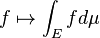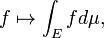# LINIARITY INTEGRAL

The collection of Riemann integrable functions on a closed interval [a, b] forms a vector space under the operations of pointwise addition and multiplication by a scalar, and the operation of integrationis a linear functional on this vector space. Thus, firstly, the collection of integrable functions is closed under taking linear combinations; and, secondly, the integral of a linear combination is the linear combination of the integrals,Similarly, the set of real-valued Lebesgue integrable functions on a given measure space E with measure μ is closed under taking linear combinations and hence form a vector space, and the Lebesgue integralis a linear functional on this vector space, so thatMore generally, consider the vector space of all measurable functions on a measure space (E,μ), taking values in a locally compact complete topological vector space V over a locally compact topological field K, f : EV. Then one may define an abstract integration map assigning to each function f an element of V or the symbol

,that is compatible with linear combinations. In this situation the linearity holds for the subspace of functions whose integral is an element of V (i.e. “finite”). The most important special cases arise when K is R, C, or a finite extension of the field Qp of p-adic numbers, and V is a finite-dimensional vector space over K, and when K=C and V is a complex Hilbert space.
Linearity, together with some natural continuity properties and normalisation for a certain class of “simple” functions, may be used to give an alternative definition of the integral. This is the approach of Daniell for the case of real-valued functions on a set X, generalized by Nicolas Bourbaki to functions with values in a locally compact topological vector space. See (Hildebrandt 1953) for an axiomatic characterisation of the integral.